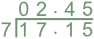Dividing Decimals

# Dividing Decimals

GCSE(F),

When dividing a decimal by an integer, use either a short division or long division method, making sure that the decimal point on the answer line is above the decimal point for the number being divided.

If the number does not divide exactly, add zeroes to the end of the number being divided to obtain the required accuracy: 95.60 is the same as 95.6.

Dividing a number by a decimal should be avoided. A division can be written as a fraction with the divisor as the denominator: multiply both numbers by 10, 100 or 1000 to remove the decimal from the denominator.  Multiplying both the numerator and the denominator by the same amount gives an equivalent fraction.

## Examples

1. Calculate 17.15 ÷ 7.frac(34)(0.08) = frac(3400)(8) = 425